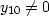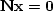Next: NMO with linear interpolation Up: NORMAL MOVEOUT AND OTHER Previous: Pseudoinverse to nearest-neighbor NMO

## Null space and inconsistency

The normal-moveout transformation is a textbook example of some of the pathologies of simultaneous equation solving. Reexamine equation (17), thinking of it as a set of simultaneous equations for xi given yi. First, (17) shows that there may exist a set of yi for which no solution xi is possible--any set containing, for example. This is an example of inconsistency in simultaneous equations. Second, there arevectors that satisfy,so any number of such vectors can be added into any solution and it remains a solution. These solutions are called the ``null space." Here these solutions are the arbitrary values of x1, x2, x3, x4, and x5 that obviously leaveunaffected. Typical matrices disguise their inconsistencies and null spaces better than does the NMO transformation. To make such a transformation, we could start from the NMO transformation and apply any coordinate transformation to the vectorsand.

## EXERCISES:

1. A succession of normal-moveout operators is called ``cascaded NMO.''

Consider NMO from time t'' to traveltime depth t' by t''2= t'2 + x2/v22, followed by another NMO transform which uses the transformation equation t'2= t2 + x2/v12. Show that the overall transformation is another NMO transformation. What is its velocity? Notice that cascaded NMO can be used to correct an NMO velocity. Thus it can be called residual velocity analysis or residual normal moveout.Next: NMO with linear interpolation Up: NORMAL MOVEOUT AND OTHER Previous: Pseudoinverse to nearest-neighbor NMO
Stanford Exploration Project
10/21/1998